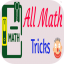Hlavní navigace

#All Math Tricks 1.0

3

Pro hodnocení programu se prosím nejprve

Staženo
1 ×
Zdarma

## Sdílet

This program is designed for those who want to learn interesting mathematical tricks to speed up the calculating. These tricks will help solve part of the mathematical problems and tasks much faster than classical. Will also be helpful to those who want to hone basics such as the multiplication table.

When you learn these mathematical tricks, you'll be able to show off your skills to friends and prove to them that you have a talent for mathematics. New skills you can use in the store, at school, at college, at work - wherever thanks to quick calculation skills can save a lot of precious time.

Fun Math Tricks:
2. Subtraction
3. Multiplication table
4. Division
5. Multiply two digit number by 11
6. Square numbers ending in 5
7. Multiply by 5
8. Multiply by 9
9. Multiply by 4
10. Dividing by 5
11. Subtracting from 1000
12. Tough multiplication
13. Power of two
14. Adding numbers close to hundreds
15. Subtracting numbers close to hundreds
16. Multiply numbers between 11 and 19
17. Square numbers between 11 and 99
18. Multiply two digit numbers having same tens digit and ones digits add up to ten
19. Square numbers between 50 and 59
20. Square numbers between 40 and 49
21. Multiply two digit numbers editing in 1
22. Percent
23. Square numbers between 100 and 109

Math Tricks Workout brings you the most entertaining practices and exercises to test and enhance your math skills. It is the best free and cool math learning app ever! Our app will let you master math and learn many math tricks to improve your skills in a very easy, fun and amazing way while surfing over the greatest brain challenging math learning app.

? EDUCATIONAL MATH GAMES
This addictive learn math app is designed for adults, students over 10 years and also for users who are preparing for competitive exams. Inspired from Vedic maths, this math workout app contains some amazing mental math and Vedic maths tricks that will help you learn math in a visual way. It contains a series of tasks to workout math tricks and test your math skills to see if you will be able to find the correct answers of each math equation. This brain training app will also help students a lot to improve their math skills by enhancing their speed and accuracy.

➗ HAVE FUN WITH MATHS ✖️
We think we have one of the best combinations of exercise math educational games. Still not convinced? Here is why you’ll want to download and install Math Tricks Workout on your android smartphone or tablet instead of other learn math games:
✅ Beautiful HD Graphic, Amazing colourful and gameplay that will give you a very great experience while learning math tricks and improving your skills.
✅ Our brain challenging app is Free and it will stay free for life so there's no fees, no special memberships and no annual subscription fees to learn math.
✅ We guarantee that you will be able to improve your math skills easily and quickly with this math workout app. We guarantee also that you will learn new skills and tricks.
✅ Math Tricks Workout - Mental maths brain training is a daily brain challenging game and it can be played by Adults and Teens. Your whole family can play it and they will enjoy it.
✅ The Speed in performing inside our app will help students and adults to learn and adopt more complicated equations.

➕LEARN MATH TRICKS➖
We think that our maths practice and exercise app is the most versatile app where you can train your brain and learn tricks! Here is a list of some of the fun math tricks that you can learn from this maths app:
• Multiplication by eleven
• Squaring of numbers ending in five
• Multiplying two numbers whose last digits add to 10
• Multiplying numbers close to 100
• Addition and subtraction of two and three digit numbers from left to right
• Mentally dividing a number by a single digit
• Multiplying two and three digit numbers using the vertical and crosswise technique
• Square two and three digit numbers using the square duplex technique

1.0

5. 10. 2017

0 ×

Ano

• #### Podporované jazyky

• Angličtina
Zobrazit více
3

Pro hodnocení programu se prosím nejprve

Staženo
1 ×